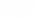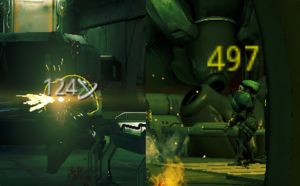（英文名：critical）## 暴击几率

 暴击几率：任意给定攻击造成额外暴击伤害的几率。数值超过100%有几率会变为橙色暴击，超过200%有几率会变为红色暴击。 –游戏内描述

= 0.675 = 67.5%

= 0.45 = 45%

= 0.75 = 75.00%

### 暴击几率计算

• 即重击时暴击几率加成翻倍的仅有斩铁和紫卡。
• 其他暴击几率加成：指武器上装备的所有MOD的暴击几率和其他与Mod算法同级的暴击几率加成（例如的被动技能）。
• 连击倍率：该部分仅限于近战武器，详见近战连击

## 暴击倍率

• 武器基础暴击倍率: 大多数的武器的固有暴击倍率2.0x。
• MOD的暴击倍率增益: 该增益为当前所装备的所有MOD带来的暴击倍率增益总和。

= 4.48

## 暴击等级

x = 0% 没有攻击能够暴击 Tier 0
0% < x < 100% 有几率触发 暴击
Tier 1
x = 100% 所有攻击均能触发黄色暴击
100% < x < 200% 有几率触发强力暴击
Tier 2
x = 200% 所有攻击均能触发橙色暴击
200% < x < 300% 有几率触发 超级暴击
Tier 3
x = 300% 所有攻击均能触发红色暴击
300% < x < 400% 有几率触发红色暴击(tier 4)
Tier 4
x = 400% 所有攻击均能触发红色暴击(tier 4)
400% < x < 500% 有几率触发 红色暴击 (tier 5)
...
... ...

• 当武器的暴击几率在100%以上，200%以下，那么暴击时有概率造成橙色暴击，能够造成比一般暴击要造成更多的伤害，并且显示伤害数值的颜色为橙色。
• 当武器的暴击几率在200%以上，300%以下，那么暴击时有概率造成红色暴击，能够造成比一般暴击要造成更多的伤害，并且显示伤害数值的颜色为红色。
• 当武器的暴击几率超过300%的时，所有暴击都将以红色字样出现。暴击几率 概率达到的暴击等级 保底达到的暴击等级 0.01% − 100% 1 N/A 100.01% − 200% 2 1 200.01% − 300% 3 2 300.01% − 400% 4 3 400.01% − 500% 5 4 500.01% − 600% 6 5 600.01% − 700% 7 6 700.01% − 800% 8 7 ••• ••• ••• n% < 暴击几率 ≤ (n% + 100%) (n% + 100%) ÷ 100 (n% + 100%) ÷ 100-1

• 超过300%暴击几率以后出现全是红色暴击。

• 即有25%的几率造成橙色暴击，75%造成黄色暴击。

• 由公式可以得到，如果裂罅Mod使得暴击倍率低于1x，高暴击等级反而会减少暴击伤害，如果武器总暴击倍率小于等于0.5x，暴击等级为2的时候，实际暴击倍率就为0了。
• 私法组合MOD有概率使得攻击的暴击等级+1。

## 部位倍率• 部位倍率不一定都为大于1的值，少部分敌人的部位拥有“抗性”，比如远古单位身后的皇冠（倍率为0.5）。
• 部份特殊敌人如恐鸟及其变种的头部位置比较特殊，甚至拥有多个弱点部位。

• 伤害:指计算完Mod、护甲、元素抗性等得到的最终伤害。
• 弱点倍率:锁定目标+60%爆头倍率、守望者开镜增加爆头伤害等影响。但特殊武器如捕月、雷霆、弧电离子枪因特殊算法导致此项无效，此时应计算为1倍。
• 实际暴击倍率:即上文中经过计算过暴击等级后的暴击倍率。

## 平均伤害

1 + 30% × (2.0 - 1) = 1.3x
• 因此，对于没有安装Mod的帕里斯，其平均暴击倍率为1.3x。（暴击倍率为2x）
1 + (30% × (1 + )) × (2.0 × (1 + ) - 1) = 3.55x
• 安装了2个暴击mod的后，其平均暴击倍率变为了3.55×。（暴击倍率为4.4x）

## 暴击伤害潜力

### 对伤害潜力的影响

• 不爆头平均伤害 = [ 暴击几率 × （暴击倍率 - 1） + 1 ] × 伤害为什么？这里是证明
• 爆头平均伤害 = 爆头倍率 × [ 暴击几率 × （暴击倍率 × 2 - 1） + 1 ] × 伤害

• 平均伤害 = 爆头几率 × 爆头平均伤害 + （1 - 爆头几率）× 不爆头平均伤害
• 爆头几率 根据玩家的实际射击情况进行估算，即通常一定射击次数下发生爆头的次数与射击次数的比值。

$\frac{d}{dp}(m (3 n - 1) + 1 - \frac{2mn}{1+p}) = \frac{2mn}{(1+p)^2}$

### 暴击相关MOD的增益

• czm: 暴击区倍率。
• p: 爆头几率，1.00表示每发均爆头，0表示没有一发爆头。
• m: 当前暴击几率，基础暴击几率 × (1 + 暴击几率加成)。
• n: 当前暴击倍率，基础暴击倍率 × (1 + 暴击倍率加成)。
• v: 当前暴头倍率，2 × (1 + 暴头倍率加成)。

### 公式证明

$m = mod(x)$ 几率造成第 $n = ceil(x)$ 级暴击

$=y(m + n - 1) - (m + n - 1) + 1$

$=xy - x + 1$

$=x(y - 1) + 1$

## 更新历史

• 增加了橙色暴击指示！现在，当暴击发生于100%至200%区间时，显示的是橙色暴击。而当暴击发生在200%以上时，显示的才是红色暴击！这仅仅是针对暴击伤害显示的改动，不会对伤害本身有任何影响。
5.0
2人评价
0

2年
0

(n% + 100%) ÷ 100=（n+100)/10000=暴击等级？我理解错了？

2年
0

2年
0

2年
0

cr: 面板暴击倍率
cp: 面板暴击概率
crit_lvl: 暴击等级
ratio1: 运气不好的伤害倍率(未达到下一暴击等级)
ratio2: 运气好的伤害倍率(达到了下一暴击等级)

crit_lvl = floor(cp)
cp' = cp - crit_lvl
ratio1 = 1 + (cr - 1) *  crit_lvl
ratio2 = 1 + (cr - 1) * (crit_lvl + 1)
total_damage_ratio = ratio1 * (1 - cp') + ratio2 * cp'

......

......

total_damage_ratio = (cr - 1) * cp + 1

3年
0

3年
0

3年
Lysinelai
0

3年
0

黄色暴击伤害/爆头倍率/暴击爆头倍率/暴击倍率=1810/2/2/2=226.25
橙色暴击伤害/爆头倍率/暴击爆头倍率/橙色暴击倍率=3168/2/2/[2*（2-1）+1]=3168/2/2/3=264(从橙字开始公式就不对了)

3年
0

3年
Lysinelai
0

3年
0

3年
Div2009
1

3年
0

3年
Smile slime 47
0

3年
0

2年
0

3年
Div2009
0

4年